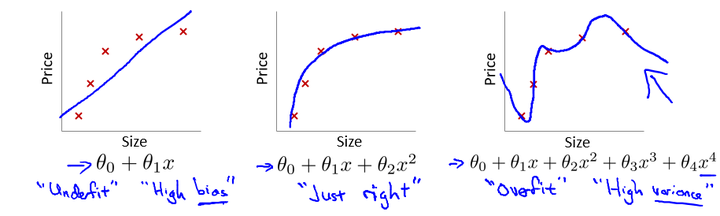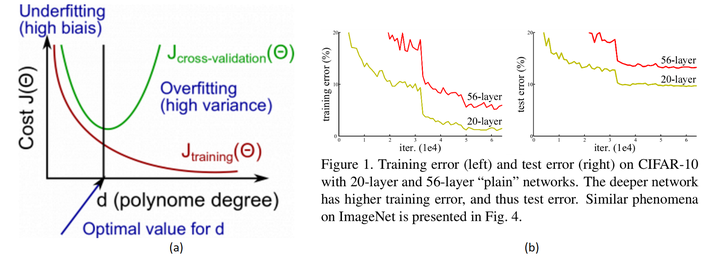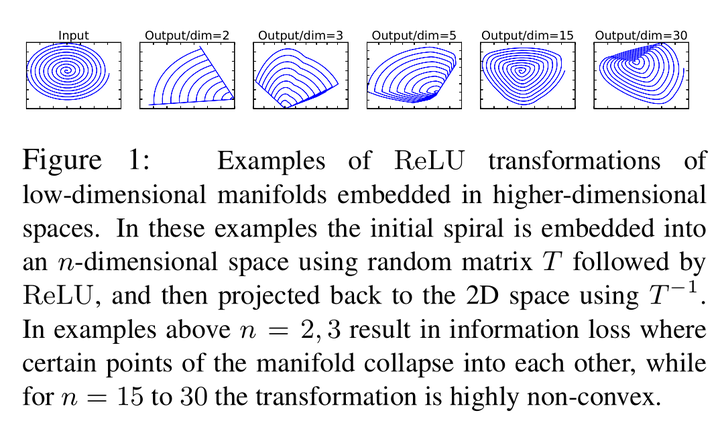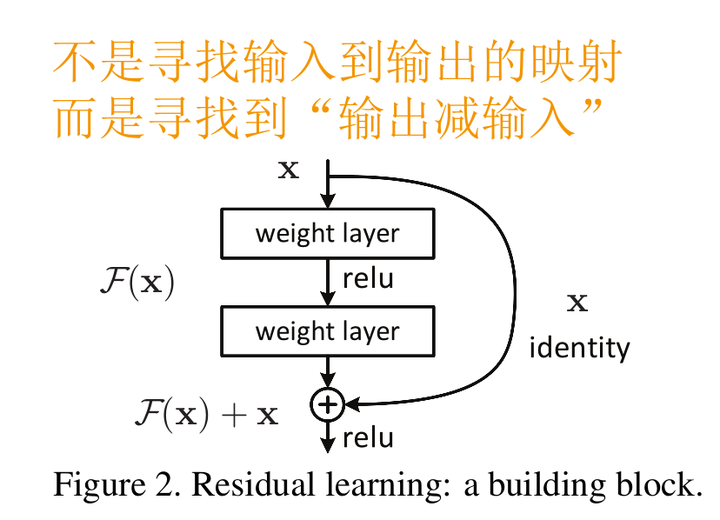# 抱歉，您的浏览器无法访问本站

### 本页面需要浏览器支持（启用）JavaScriptCIFAR-10上的训练误差（左）和测试误差（右），具有20层和56层“普通”网络。网络越深，训练误差越大，因此测试误差越大。

$$x=-2, y=5, z=-4$$带入，其中，令$$x+y=q$$，一步步计算，很容易就能得出$$f(-2,5,-4)=-12$$MobileNet V2的论文也提到过类似的现象，由于非线性激活函数Relu的存在，每次输入到输出的过程都几乎是不可逆的（信息损失）。我们很难从输出反推回完整的输入。$\mathbf{y}=\mathcal{F}\left(\mathbf{x},\left\{W_{i}\right\}\right)+\mathbf{x} \quad \rightarrow \quad \mathbf{y}=\mathcal{F}\left(\mathbf{x},\left\{W_{i}\right\}\right)+W_{s} \mathbf{x}$ 而对于深度上的不一致，则有两种解决办法，一种是在跳接过程中加一个1*1的卷积层进行升维，另一种则是直接简单粗暴地补零。事实证明两种方法都行得通。